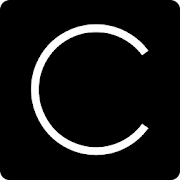CalvertersEveryone
66
We are bringing you all the important calculators and converters at single place so you don't have to download different application for your all need.

We have many unit converters in this application which you can use for different purposes in your daily life.

We have following calculators in our app.
- Root Calculator
- Discount Calculator
- Age Calculator
- Date Calculator
- Decimal to Fraction
- Percentage Calculator
- Health Calculator
- BMI Calculator
- BMR Calcualtor
- Calorie Calculator
- IBW Calculator
- LBM Calculator
- BSA Calcualtor
- Number Series Calcualtor
- Arithmetic Sequence
- Geomatric Sequence
- Fibonacci Sequence
- GCF & LCM Calculator
- Number Base
- Area Calcualtor
- Triangle
- Circle
- Rectangle
- Square
- Ellipse
- Parallelogram
- Trapezoid
- Cricle Arc
- Pentagon
- Hexagon
- Volume Calculator
- Cube
- Sphere
- Cylinder
- Cone
- Square Pyramid
- Fraction Calculator

We have following converters in our app.
- Length
- Angle
- Weight
- Time
- Digital Storage
- Temperature
- Area
- Speed
- Volume
- Pressure
- Sound
- Energy
- Force
- Power
- Fuel Consumption
- Torque
- Frequency

Please let us know if you find any problem in any calculation or any conversion. So we can update it. All the calculation here are taken from public domain.
Collapse

Review Policy
5.0
66 total
5
4
3
2
1

What's New

- added many new calculators and converters
- added new colored design for different calculators and converters
Collapse

Updated
October 21, 2017
Size
3.6M
Installs
100+
Current Version
1.1.2
Requires Android
4.2 and up
Content Rating
Everyone
Permissions
Offered By
Unified Apps
Developer